Courses

# Test: Time Varying EMT Fields- 2

## 15 Questions MCQ Test Topicwise Question Bank for Electrical Engineering | Test: Time Varying EMT Fields- 2

Description
This mock test of Test: Time Varying EMT Fields- 2 for Electrical Engineering (EE) helps you for every Electrical Engineering (EE) entrance exam. This contains 15 Multiple Choice Questions for Electrical Engineering (EE) Test: Time Varying EMT Fields- 2 (mcq) to study with solutions a complete question bank. The solved questions answers in this Test: Time Varying EMT Fields- 2 quiz give you a good mix of easy questions and tough questions. Electrical Engineering (EE) students definitely take this Test: Time Varying EMT Fields- 2 exercise for a better result in the exam. You can find other Test: Time Varying EMT Fields- 2 extra questions, long questions & short questions for Electrical Engineering (EE) on EduRev as well by searching above.
QUESTION: 1

Solution:
QUESTION: 2

### A circular coil begins completely outside any magnetic field, and then during the next 10 sec. it moves completely into a uniform 15T magnetic field. Assuming that the plane of the loop and the direction of motion are both perpendicular to the magnetic field, the magnitude of induced emf in the coil with 25 loops and radius of 7 cm is

Solution:

Since the loop begins outside of any magnetic field, therefore initial magnetic flux is zero.
The final flux is given by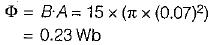Using Faraday’s law, emf induced in the loop is given by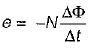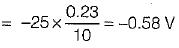∴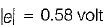QUESTION: 3

### A 18 cm conducting rod attached to a U-shaped conductor moves at a speed of 1 cm/s perpendicular to a magnetic field of strength 37.5T as shown in figure below.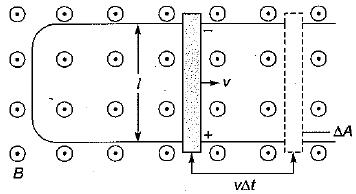What is the emf induced in the conducting rod?

Solution:

The motional emf induced when the conductor’s velocity, length and the magnetic field are all perpendicular is given by
e = BIv
= 37.5 x 0.18 x 0.01
= 0.07 V

QUESTION: 4

What is the strength of the electric field produced in the conductor by the change in flux in the above problem?

Solution:

The electric field produced is given by
E = vB = 0.01 x 37.5
= 0.375 N/C = 0.375 V/m
or, E = 0.38 N/C or V/m

QUESTION: 5

What is the induced emf produced when the radius of one loop or wire in a 12T magnetic field changes from 2 cm to 9 cm in 3 seconds?
Assume that the plane of the loop remains parallel to the direction of the magnetic field.

Solution:

An emf is not induced if the plane of the coil is parallel to the lines of the magnetic field since the magnetic flux through the coil does not change when the coil shrinks.

QUESTION: 6

When a magnetic flux cuts across 200 turns at the rate of 2 Wb/s, the induced voltage is

Solution:

Induced emf,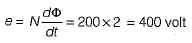QUESTION: 7

A conducting bar can slide freely over two conducting rails as shown in figure.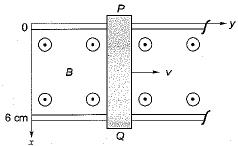What is the magnitude of maximum induced voltage in the bar if the bar is stationed at y = 8 cm and B = 4 cos 106 t azmWb/m2?

Solution:

The transformer emf is given by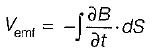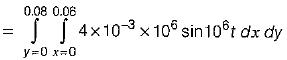= 19.2 sin 106 t V
∴ |Vemf|max = 19.2V

QUESTION: 8

A parallel plate capacitor with plate area of 5 cm2 and plate separation of 3 mm has a voltage 50sin103t applied to its plate. Calculate the displacement current assuming ε = 2ε0.

Solution: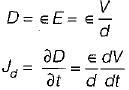(S → Area)
Here,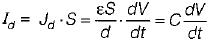Which is the same as the conduction current given by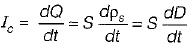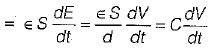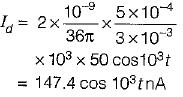QUESTION: 9

The flux through each turn of a 100-turn coil is (t3 - 2t) mWb, where t is in seconds. The induced emf at t = 2 sec. is

Solution:

Given,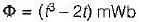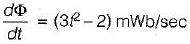Emf induced is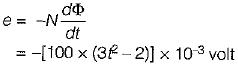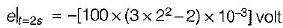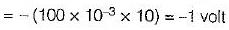QUESTION: 10

A loop is rotating about the y-axis in a magnetic field B = (B0 sinωt) ax mWb/m2. The voltage induced in the loop is due to

Solution:
QUESTION: 11

The concept of displacement current is a major contribution attributed to

Solution:
QUESTION: 12

Which of the following expressions does not represent Maxwell’s equations for time-varying fields?

Solution:
QUESTION: 13

An electromagnetic field is said to be conservative when

Solution:

∇ x E  = 0 for conservative field.

QUESTION: 14

A loop is inside a uniform magnetic field of B = 50 ax mWb/m2 as shown in figure. If side DC of the loop cuts the flux lines at the frequency of 50 Hz and the loop lies in the yz plane at time t = 0, what is the induced emf at t = 5 ms?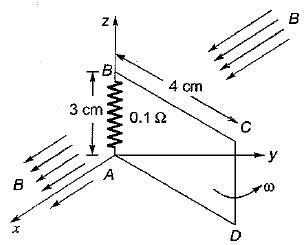Solution: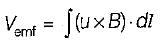Given, dl = dlDC = dZaz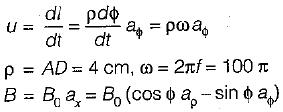and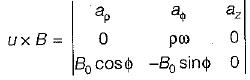= -ρωB0 cos ϕ az
Now, (u x B)·dl = -ρB0ω cos ϕ dz
= - 0.2 π cos ϕ dz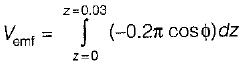= -6π cos ϕ mv
Also, Vemf = - 6π cos (ωt + π/2), at t = 5 ms
∴  |Vemf| = 6 πmV

QUESTION: 15

If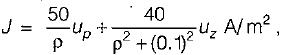the total current crossing the plane z = 0.2 in the uz direction for p < 0.4 is

Solution: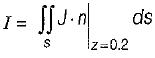or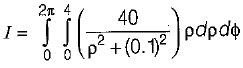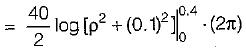or, I = 40π log 17 = 356 A